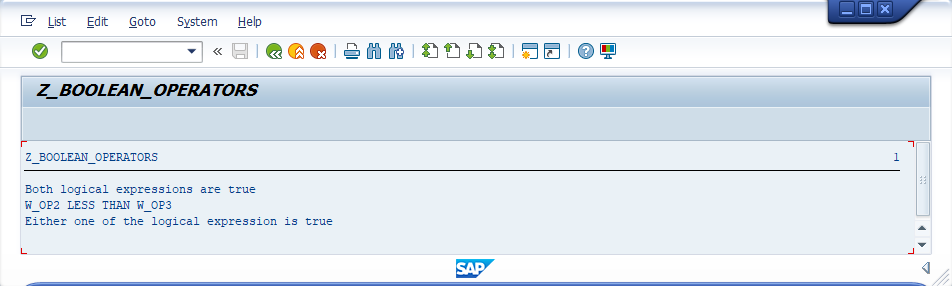###### Summary -

In this topic, we described about the below sections -

Boolean Operators are used to combine the logical expressions to produce a single output. Logical expression is a combination of one or more operands with a relational operator.

Logical expression output is either true or false. Below are the list of Boolean operators -

OperatorDescriptionSyntax
NOTNegotiation of logical expression with NOTNOT log_exp
ANDTrue when all the logical expressions are combined with AND are truelog_exp1 AND log_exp2 AND log_exp3 ...
EQUIVTrue when all the logical expressions are combined with EQUIV are true or falselog_exp1 EQUIV log_exp2 ...
ORTrue when all the logical expressions are combined with OR are true or any one of the logical expression is truelog_exp1 OR log_exp2 OR log_exp3 ...

Below table describes how the AND, OR, XOR and NOT operations worked on boolean operators -

Logical Expression (LE1)Logical expression (LE2)LE1 AND LE2LE1 OR LE2LE1 EQUIV LE2NOT LE1NOT LE2
FalseFalseFalseFalseTrueTrueTrue
FalseTrueFalseTrueFalseTrueFalse
TrueFalseFalseTrueFalseFalseTrue
TrueTrueTrueTrueTrueFalseFalse

#### Example -

Below example shows how the Boolean operations coded in the program.

#### Code -

``````*&---------------------------------------------------------------------*
*& Report  Z_BOOLEAN_OPERATORS
*&---------------------------------------------------------------------*
*& Written by TutorialsCampus
*&---------------------------------------------------------------------*

REPORT  Z_BOOLEAN_OPERATORS.

* Declaring Variables
DATA: W_OP1 TYPE I VALUE 40,
W_OP2 TYPE I VALUE 60,
W_OP3 TYPE I VALUE 80.

* Logical AND expression
IF ( W_OP1 LT W_OP2 ) AND ( W_OP2 LT W_OP3 ).
WRITE 'Both logical expressions are true'.
ELSE.
WRITE 'Both logical expressions are not true'.
ENDIF.

* Logical NOT operator
IF NOT ( W_OP2 GT W_OP3 ).
WRITE /'W_OP2 LESS THAN W_OP3'.
ENDIF.

* Logical OR operator
IF ( W_OP1 LT W_OP2 ) OR ( W_OP2 GT W_OP3 ).
WRITE /'Either one of the logical expression is true'.
ELSE.
WRITE /'Neither one of the logical expression is true'.
ENDIF.
``````

#### Output -#### Explaining Example -

In the above example, each and every statement is preceeded with a comment to explain about the statement. Go through them to get clear understanding of example code.

IF ( W_OP1 LT W_OP2 ) AND ( W_OP2 LT W_OP3 ), verifies ( W_OP1 LT W_OP2 ) and ( W_OP2 LT W_OP3 ) are true or not. If true, displays 'Both logical expressions are true'. otherwise, 'Both logical expressions are not true' gets displayed.

IF NOT ( W_OP2 GT W_OP3 ), verifies ( W_OP2 GT W_OP3 ) expression output is false not. If false, displays 'W_OP2 LESS THAN W_OP3'.

IF ( W_OP1 LT W_OP2 ) OR ( W_OP2 GT W_OP3 ), verifies either ( W_OP1 LT W_OP2 ) or ( W_OP2 GT W_OP3 ) are true or not. If true, displays 'Either one of the logical expression is true'. otherwise, 'Neither one of the logical expression is true' gets displayed.